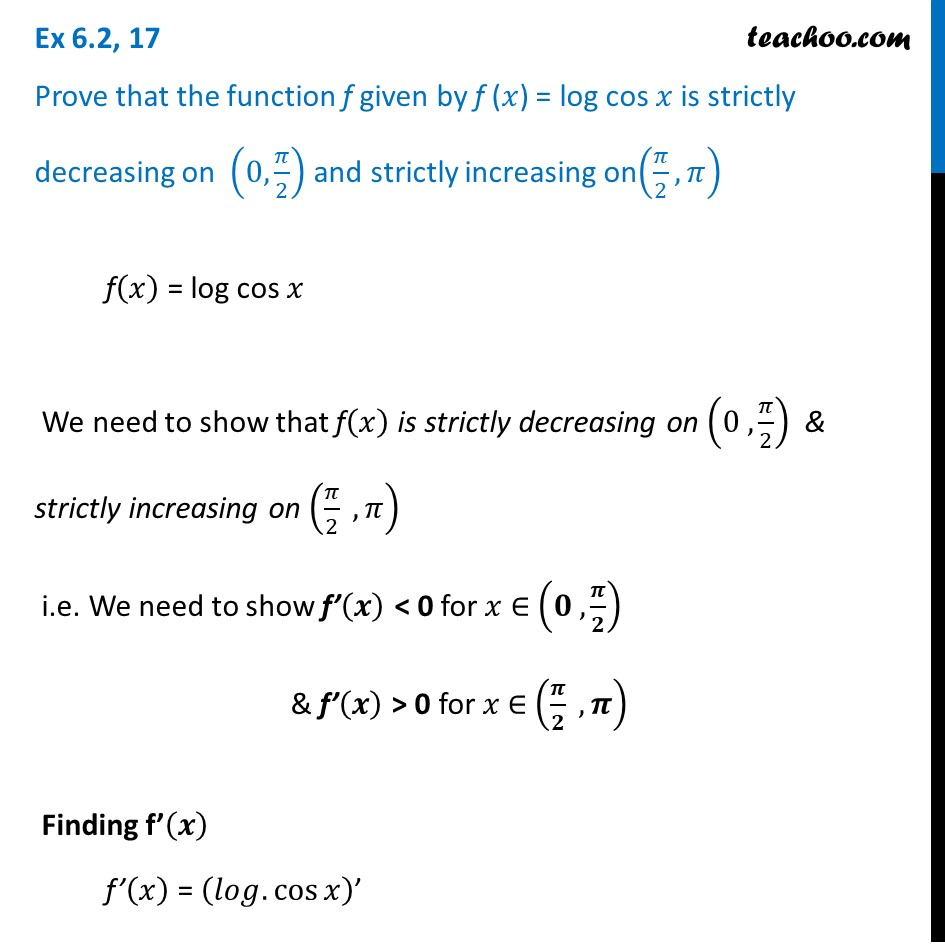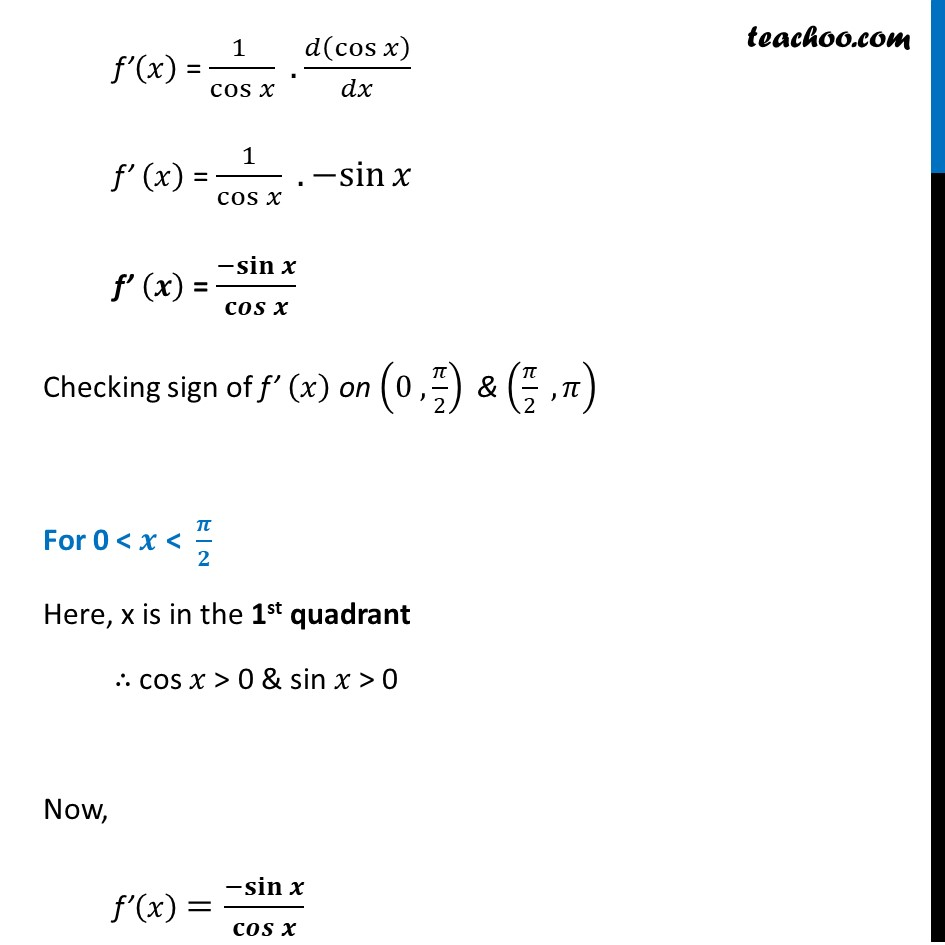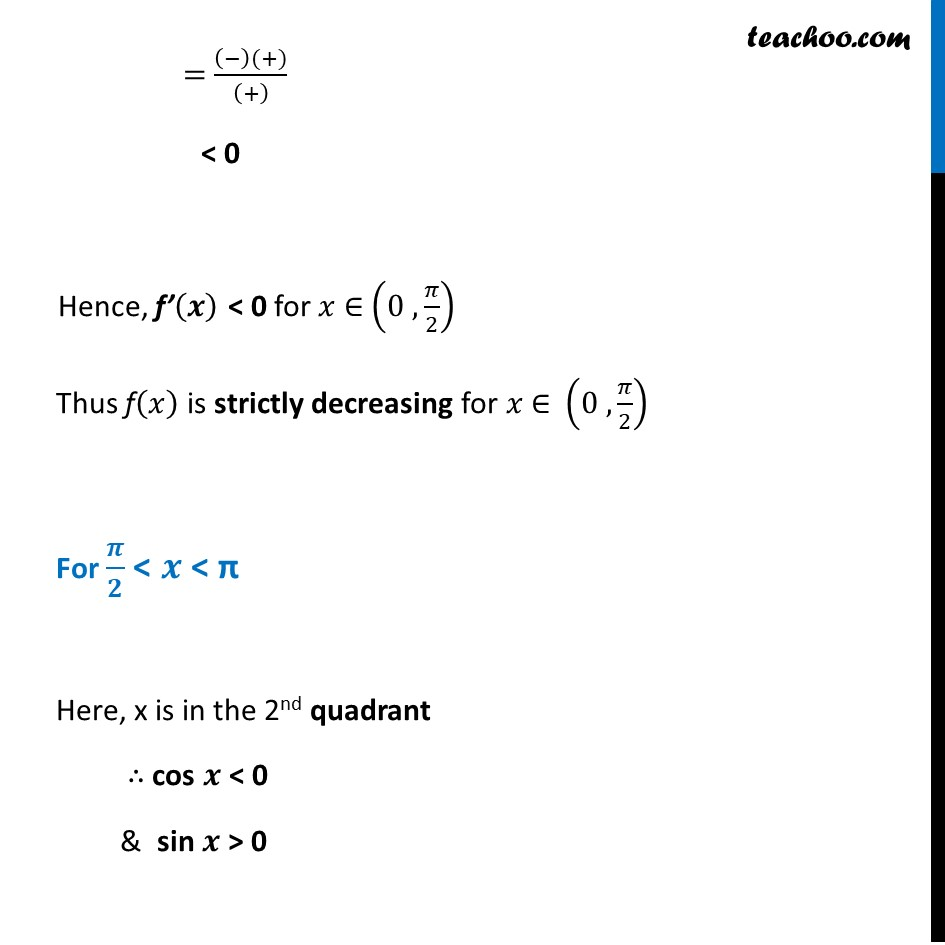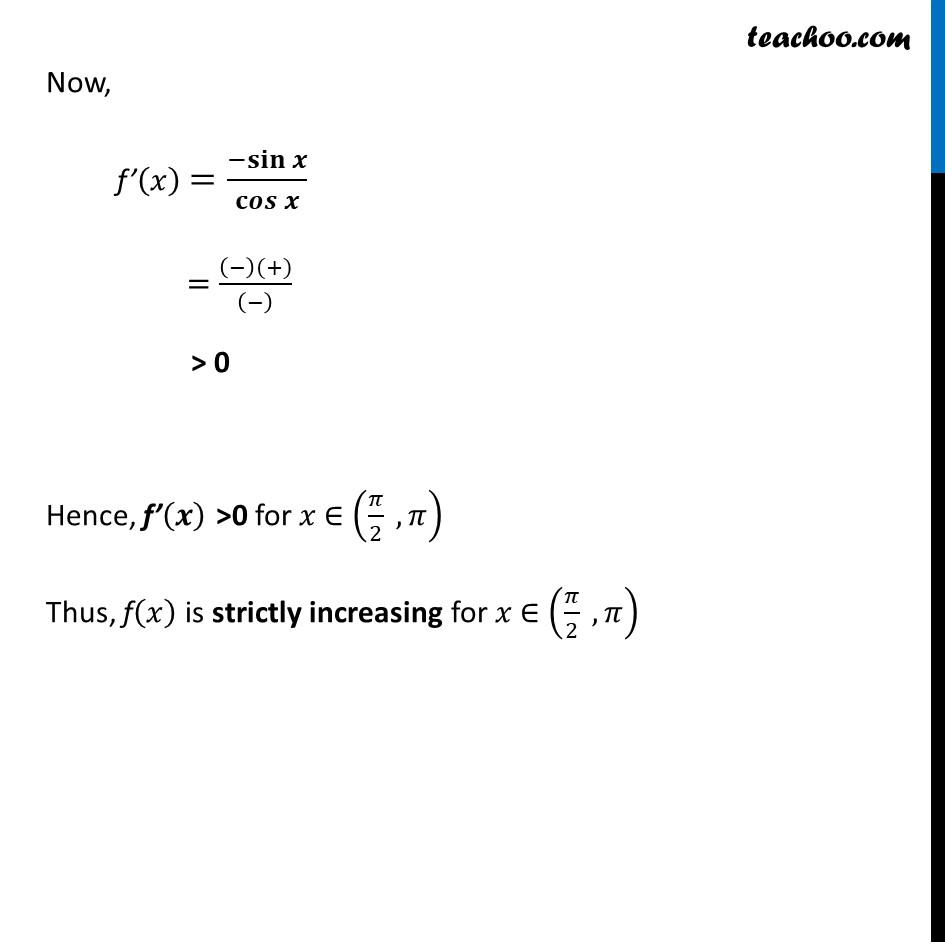Ex 6.2

Chapter 6 Class 12 Application of Derivatives
Serial order wiseGet live Maths 1-on-1 Classs - Class 6 to 12

### Transcript

Ex 6.2, 17 Prove that the function f given by f (𝑥) = log cos 𝑥 is strictly decreasing on (0,𝜋/2) and strictly increasing on(𝜋/2,𝜋) f(𝑥) = log cos 𝑥 We need to show that f(𝑥) is strictly decreasing on (0 , 𝜋/2) & strictly increasing on (𝜋/2 , 𝜋) i.e. We need to show f’(𝒙) < 0 for 𝑥 ∈ (𝟎 , 𝝅/𝟐) & f’(𝒙) > 0 for 𝑥 ∈ (𝝅/𝟐 , 𝝅) Finding f’(𝒙) f’(𝑥) = (𝑙𝑜𝑔.cos⁡𝑥 )’ f’(𝑥) = (1 )/cos⁡𝑥 . 𝑑(cos⁡𝑥 )/𝑑𝑥 f’ (𝑥) = 1/cos⁡𝑥 .〖−sin〗⁡𝑥 f’ (𝒙) = 〖−𝐬𝐢𝐧〗⁡𝒙/𝐜𝒐𝒔⁡𝒙 Checking sign of f’ (𝑥) on (0 , 𝜋/2) & (𝜋/2 , 𝜋) For 0 < 𝒙 < 𝝅/𝟐 Here, x is in the 1st quadrant ∴ cos 𝑥 > 0 & sin 𝑥 > 0 Now, f’(𝑥) =〖−𝐬𝐢𝐧〗⁡𝒙/𝐜𝒐𝒔⁡𝒙 = ((−)(+))/((+) ) < 0 Hence, f’(𝒙) < 0 for 𝑥 ∈ (0 , 𝜋/2) Thus f(𝑥) is strictly decreasing for 𝑥 ∈ (0 , 𝜋/2) For 𝝅/𝟐 < 𝒙 < π Here, x is in the 2nd quadrant ∴ cos 𝒙 < 0 & sin 𝒙 > 0 Now, f’(𝑥) =〖−𝐬𝐢𝐧〗⁡𝒙/𝐜𝒐𝒔⁡𝒙 = ((−)(+))/((−) ) > 0 Hence, f’(𝒙) >0 for 𝑥 ∈ (𝜋/2 , 𝜋) Thus, f(𝑥) is strictly increasing for 𝑥 ∈ (𝜋/2 , 𝜋)